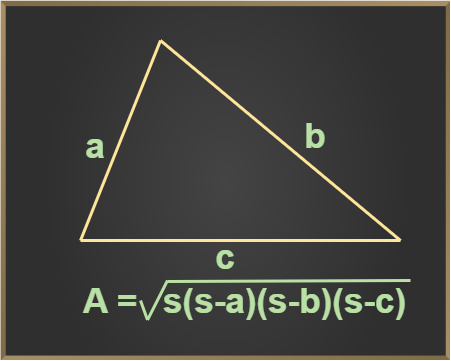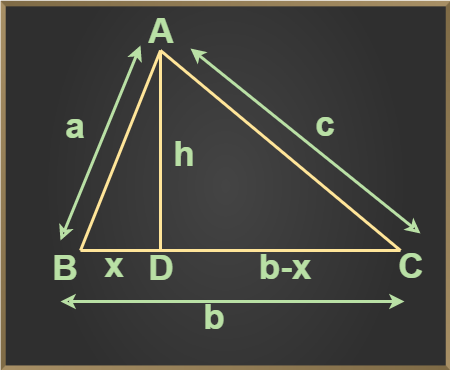Open in App
Not now

# Heron’s Formula

• Last Updated : 20 Feb, 2023

Heron’s Formula is a very popular formula for finding the area of a triangle when the three sides are given. This formula was given by “Heron” in his book “Metrica”. We can apply this formula to all types of triangles, be they right-angled, equilateral, or isosceles. Let’s learn about Heron’s formula and its derivation in detail.

## What is Heron’s Formula?

Heron’s formula is helpful in determining the area of a triangle if the value of the length of all the sides of the triangle is given. Heron’s formula is also used for finding the area of a quadrilateral as a quadrilateral can easily be divided into two triangles by the diagonals of the quadrilateral.  According to this formula, the area of a triangle is directly proportional to the square root of the semi-perimeter of the triangle. If the sides of the triangle are a, b, and c then Heron’s Formula is,

A = √{s(s-a)(s-b)(s-c)}

where,
A is area of Triangle ABC
a, b, c are lengths of the sides of the triangle
s is semi-perimeter = (a + b + c) / 2

## History of Heron’s Formula

In 60 CE Heron of Alexandria gave a formula which is known as the Heron’s Formula. Heron was a Greek Mathematician who gave the formula for finding the area of a triangle when the length of all sides of the triangle is given.

## Heron’s Formula Definition

Heron’s formula, states that, “For a triangle having lengths, a, b, and c, and semi-perimeter of the triangle is ‘s’ the area of the triangle using Heron’s Formula” is,## Proof for Heron’s Formula

Heron’s formula is derived using Pythagoras Theorem, the Area of a Triangle, and others. For a triangle having lengths of sides, a, b, and c. The semi-perimeter of the triangle ABC is “s”,

s = (a + b + c) / 2

### Heron’s Formula Derivation

The proof for Heron’s Formula is discussed in the article below

Area of a Triangle = (1/2) × b × h

where,
b is the base
h is the heightDraw a perpendicular AD on BC

From the ∆ ABD,

a2 = x2 + h2

x2 = (a2−h2)….(i)

x = √(a2−h2)….(ii)

Consider the  ∆ACD,

(b−x)2 + h2 = c2

(b−x)2 = c2 − h2

b2 − 2bx + x2 = c2–h2

Putting the value of x and x2 from equations (i) and (ii) in the above equation, we get

b2 – 2b√(a2−h2)+ a2−h2 = c2 − h2

b2 + a2 − c2 = 2b√(a2 − h2)

Squaring on both sides, we get;

(b2 + a2 – c2)2 = 4b2(a2−h2)

{(b2 + a2 – c2)2) / 4b2 = (a2−h2)

a2 + {(b2 + a2 – c2)2) / 4b2 = h2

simplifying, we get

h2 = (a+b+c)(b+c-a)(a+c-b)(a+b-c) / 4b2

Now, 2s = a+b+c, where s is the semi-perimeter of the triangle.

h2 = 2s(2s-2a)(2s-2b)(2s-2c) / 4b2

h = √[2s(2s-2a)(2s-2b)(2s-2c)] / 2b

h = 2×√[s(s-a)(s-b)(s-c)] / b…(iii)

From, area of triangle = 1/2 × b × h

Now, area of triangle = 1/2 × {b × 2×√[s(s-a)(s-b)(s-c)]} / b

Area of Triangle (A) = √[s(s-a)(s-b)(s-c)]

## How to Find Area Using Heron’s Formula?

Follow the following steps to find the area of a triangle using Heron’s formula

Step 1: Calculate the perimeter of the given triangle

Step 2: Divide the value of the perimeter by 2 to get the semi-perimeter of the given triangle; S = (a+b+c)/2

Step 3: Use Heron’s formula A = √(s(s – a)(s – b)(s – c) to find the area of the triangle.

Thus, the area of the triangle is found.

## Heron’s Formula for Equilateral Triangle

For an equilateral triangle, all sides are equal. Now, the semi-perimeter of the equilateral triangle is

s = (a+a+a) / 2

s = 3a / 2

where a is the length of the side.

Now, using Heron’s Formula,

Area of Equilateral Triangle = √(s(s – a)(s – a)(s – a)

Area of Equilateral Triangle = √3 / 4 × a2

Also, Check

## Solved Examples on Heron’s Formula

Example 1: Calculate the area of a triangle whose lengths of sides a, b, and c are 14cm,13cm, and 15 cm respectively.

Solution:

Given:

a = 14cm
b = 13cm
c = 15cm

Firstly, we will determine semi-perimeter(s)

s = (a + b + c)/2

s = (14 + 13 + 15)/2
s = 21 cm

A = √(s(s – a)(s – a)(s – a)

A = √(21(21 – 14)(21 – 13)(21 – 15)

A = 84 cm2

Example 2: Find the area of the triangle if the length of two sides is 11cm and 13cm and the perimeter is 32cm.

Solution:

Let a, b and c be the three sides of the triangle.

a = 11cm
b= 13 cm

c = ?

Perimeter = 32cm

As we know, Perimeter equals to the sum of the length of three sides of a triangle.

Perimeter = (a + b + c)

32 = 11 + 13 + c

c = 32 – 24

c= 8 cm

Now as we already know the value of perimeter,

s = perimeter / 2

s = 32 / 2

s =16 cm

a = 11cm, b = 13 cm, c = 8 cm, s = 16 cm

A = √(s(s – a)(s – a)(s – a)

A = √(16(16 – 11)(16 – 13)(16 – 8)

A = 43.8 cm2

Example 3: Find the area of an equilateral triangle with a side of 8 cm.

Solution:

Given,

Side = 8 cm

Area of Equilateral Triangle = √3 / 4 × a2

Area of Equilateral Triangle = √3 / 4 × (8)2

= 16 √3 cm2

## FAQs on Heron’s Formula

### Question 1: What is Heron’s Formula?

Heron’s Formula is the formula to find the area of a triangle when its three sides are given,

A = √{s(s-a)(s-b)(s-c)}

### Question 2: Who gave Heron’s formula?

Heron’s formula was given by Hero of Alexandria a great mathematician of ancient Greece.

### Question 3: What does ‘s’ represent in Heron’s Formula?

‘s’ in Heron’s formula represents the semi-perimeter of the triangle which is calculated as,

s = (a+b+c)/2

where
a, b and c are three sides of a triangle.

### Question 4: When is Heron’s formula used?

We use Heron’s formula for finding the side of the triangle when all its three sides are known.

### Question 5: What is Heron’s formula for an equilateral triangle?

Area of an equilateral triangle with side ‘a’ can be found using the formula,

A = √3 / 4 × a2

### Question 6: How can we find the area of the quadrilateral using Heron’s formula?

Area of the quadrilateral is found using Heron’s formula by following the steps,

Step 1: Divide the quadrilateral into two triangles using their diagonals.

Step 2: Find the area of both triangles individually using Heron’s formula.

Step 3: Add both the areas of the triangle to get the area of the quadrilateral.

My Personal Notes arrow_drop_up
Related Articles